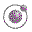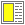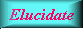Name binding, Recursion, Iteration, and Continuations - slide 6 : 42

An example with let*
```(define (how-many-days-hours-minutes-seconds n)
(let* ((days     (quotient n seconds-in-a-day))
(n-rest-1 (modulo n seconds-in-a-day))
(hours    (quotient n-rest-1 seconds-in-an-hour))
(n-rest-2 (modulo n-rest-1 seconds-in-an-hour))
(minutes  (quotient n-rest-2 60))
(seconds  (modulo n-rest-2 60))
)
(list days hours minutes seconds)))```A set of functions for handling of time.